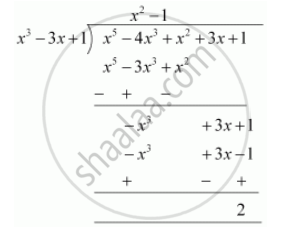# Check whether the first polynomial is a factor of the second polynomial by dividing the second polynomial by the first polynomial x^3 – 3x + 1, x^5 – 4x^3 + x^2 + 3x + 1 - Mathematics

Check whether the first polynomial is a factor of the second polynomial by dividing the second polynomial by the first polynomial

x3 – 3x + 1, x5 – 4x3 + x2 + 3x + 1

Check whether the first polynomial is a factor of the second polynomial by applying the division algorithm g(x) = x3 – 3x + 1, f(x) = x5 – 4x3 + x2 + 3x + 1

#### Solution

x3 - 3x + 1, x5 - 4x3 + x2 + 3x + 1Since the remainder ≠ 0

Hence x3 - 3x + 1 is not a factor of x5 - 4x3 + x2 + 3x + 1

Concept: Division Algorithm for Polynomials
Is there an error in this question or solution?
Chapter 2: Polynomials - Exercise 2.3 [Page 36]

#### APPEARS IN

NCERT Class 10 Maths
Chapter 2 Polynomials
Exercise 2.3 | Q 2.3 | Page 36
RD Sharma Class 10 Maths
Chapter 2 Polynomials
Exercise 2.3 | Q 2.2 | Page 57

Share